# Binomial probability

What is the binominal probability that at least 4 of the 6 trials (n=6) are succeeded where φ = 0.50?

p =  0.3438

### Step-by-step explanation: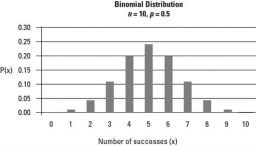Did you find an error or inaccuracy? Feel free to write us. Thank you!Tips to related online calculators
Looking for a statistical calculator?
Would you like to compute count of combinations?

## Related math problems and questions:

• DiceWe throw five times the dice. What is the probability that six fits exactly twice?
• Mortality tables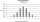Mortality tables enable actuaries to obtain the probability that a person at any age will live a specified number of years. Insurance companies and others use such probabilities to determine life-insurance premiums, retirement pensions, and annuity paymen
• Families 2Seven hundred twenty-nine families are having six children each. The probability of a girl is 1/3, and the probability of a boy is 2/3. Find the number of families having two girls and four boys.
• Doctors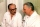The drug successfully treats 90% of cases. Calculate the probability that he will cure at least 18 patients out of 20?
• Probability of failures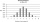In certain productions, the probability of failures is 0.01. Calculate the probability that there will be more than one failure among the 100 selected products if we return the selected products to the file after the check.
• SeedsThe germination of seeds of a certain species of carrot is 96%. What is the probability that at least 25 seeds out of 30 will germinate?
• DiceWe throw 10 times a playing dice. What is the probability that the six will fall exactly 4 times?
• Bernoulli distribution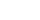The production of solar cells produces 2% of defective cells. Assume the cells are independent and that a lot contains 800 cells. Approximate the probability that less than 20 cells are defective. (Answer to the nearest 3 decimals).
• SalesFrom statistics of sales goods, item A buy 51% of people and item B buys 59% of people. What is the probability that from 10 people buy 2 item A and 8 item B?
• FamilyWhat is the probability that a family with 7 childrens have: exactly 6 dívek? 2 dívky and 5 boys? Consider the birth probability of a girl is 48.87% and boy 51.13%.
• Family94 boys are born per 100 girls. Determine the probability that there are two boys in a randomly selected family with three children.
• RecordsRecords indicate 90% error-free. If 8 records are randomly selected, what is the probability that at least 2 records have no errors?
• Probability of malaria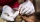A survey carried out at a certain hospital indicates that the probability that a patient tested positive for malaria is 0.6. What is the probability that two patients selected at random (i) one is negative while the other tested positive? (i) both patient
• All use computerIt is reported that 72% of working women use computers at work. Choose 3 women at random, find the probability that all 3 women use a computer in their jobs.
• Playing cards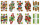From 32 playing cards containing 8 red cards, we choose 4 cards. What is the probability that just 2 will be red?
• Component fail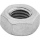There is a 90 percent chance that a particular type of component will perform adequately under high temperature conditions. If the device involved has four such components, determine the probability that the device is inoperable because exactly one of the
• Internet anywhere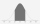In school, 60% of pupils have access to the internet at home. A group of 8 students is chosen at random. Find the probability that a) exactly 5 have access to the internet. b) At least 6 students have access to the internet